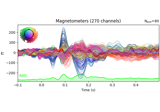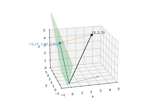# mne.compute_proj_epochs¶

mne.compute_proj_epochs(epochs, n_grad=2, n_mag=2, n_eeg=2, n_jobs=1, desc_prefix=None, meg='separate', verbose=None)[source]

Compute SSP (signal-space projection) vectors on epoched data.

This function aims to find those SSP vectors that will project out the `n` most prominent signals from the data for each specified sensor type. Consequently, if the provided input data contains high levels of noise, the produced SSP vectors can then be used to eliminate that noise from the data.

Parameters
epochsinstance of `Epochs`

The epochs containing the artifact.

n_grad`int`

n_mag`int`

Number of vectors for magnetometers.

n_eeg`int`

Number of vectors for EEG channels.

n_jobs`int`

The number of jobs to run in parallel (default 1). Requires the joblib package. Number of jobs to use to compute covariance.

desc_prefix

The description prefix to use. If None, one will be created based on the event_id, tmin, and tmax.

meg`str`

Can be ‘separate’ (default) or ‘combined’ to compute projectors for magnetometers and gradiometers separately or jointly. If ‘combined’, `n_mag == n_grad` is required and the number of projectors computed for MEG will be `n_mag`.

New in version 0.18.

verbose

If not None, override default verbose level (see `mne.verbose()` and Logging documentation for more). If used, it should be passed as a keyword-argument only.

Returns
projs: `list`

List of projection vectors.

## Examples using `mne.compute_proj_epochs`¶